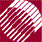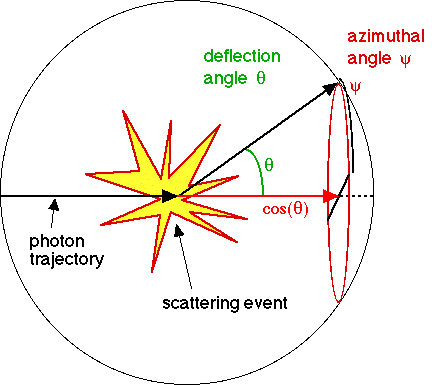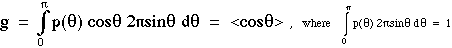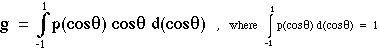ECE532 Biomedical Optics © 1998 Steven L. Jacques, Scott A. Prahl Oregon Graduate Institute

# Definition of anisotropy, g [dimensionless]

The anistoropy, g [dimensionless], is a measure of the amount of forward direction retained after a single scattering event. Imagine that a photon is scattered by a particle so that its trajectory is deflected by a deflection angle θ, as shown in the figure below. Then the component of the new trajectory which is aligned in the forward direction is shown in red as cos(θ). On average, there is an average deflection angle and the mean value of cos(θ) is defined as the anisotropy. 13A scattering event causes a deflection at angle θ from the original forward trajectory. There is also an azimuthal angle of scattering, ψ.
But it is the deflection angle θ which affects the amount of forward direction, cos(θ), retained by the photon.

Consider an experiment in which a laser beam strikes a target such as a cylindrical cuvette containing a dilute solution of scattering particles. The scattering pattern p(θ) is measured by a detector that is moved in a circle around the target while always facing the target. Hence the detector collects light scattered at various deflection angles θ in a horizontal plane parallel to the table top on which the apparatus sits. The proper definition of anisotropy is the expectation value for cos(θ):It is common to express the definition of anisotropy in an equivalent way: